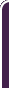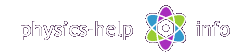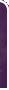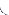homephysics online guidephysics help my info terms of use contactsWhat is Physics? Mechanics Electricity and Magnetism Electric Field Gauss' Law about below subjects Electric Potential Capacity Direct Current Magnetic Field Magnetic Field Laws Magnetic Interactions Electromagnetic Induction Maxwell's Equations Oscillations and Waves Simple Harmonic Motion Damped Harmonic Motion Driven Harmonic Motion Electric Oscillation Alternating Current Wave Motion Elastic Waves Electromagnetic Waves Optics Light Waves Geometrical Optics Interference Polarization Diffraction Fraunhofer Diffraction Dispersion, Absorption, Diffusion Doppler Effect Thermodynamics Ideal Gas Molecular Statistics Transport Phenomena First Law of Thermodynamics Second and Third Laws of Thermodynamics Imperfect Gas Liquids Solids Quantum Physics Thermal Radiation Quantum Properties of Light Wave Properties of Particles Planetary Model of Atom X-Rays Particle in Potential Well Pauli Exclusion Principle Nuclear Physics Solid State Physics Appendices# Vectors

Vector is a quantity specified by magnitude plus direction in space

## Properties of vectors

Any vectoris uniquely specified by its three components x, y, z which are projections of the vector on coordinate axes with unit vectors(such that)The notation of vector with coordinates has the form

isMagnitude of vector(or its length) is defined by Pythagorean Theorem

|| =Adding of vectorsandis sum vectorThe sum vector is determined by Parallelogram Rule of additionMagnitude of sum vector is defined by Law of Cosineswhere:andare magnitudes of the vectorsandis angle between them

Components of sum vector:

x = x1+ x2

y = y1+ y2

z = z1+ z2

The parallelogram rule of addition is partial case of general Polygon Rule used for adding several vectors## Subtracting vectors

Subtracting of vectorsandis vector differenceThe vector difference is determined by Triangle Method of subtractionMagnitude of vector differenceComponents of vector difference:

x = x1 - x2

y = y1 - y2

z = z1 - z2

## Scalar Product

Scalar (or dot) product of vectorsandis scalar quantity defined bywhereis angle between vectorsandProperties of scalar product:

1)2) Ifthen3) Ifthen4)## Vector product

Vector (or cross) product of vectorsandis vectorThe vectoris normal to plane in which the vectorsandlie (plane x-y) directed in accordance with Right-Hand Rule: when right palm is half-bent fromtoits thumb shows the direction of vectorMagnitude of vectorwhereis angle between vectorsandComponents of vector product:Properties of vector product:

1)2) Ifthen3) Ifthen magnitude of vector product ishome  |  physics online guide  |  physics help  |  my info  |  terms of use  |  contacts Dr. Nikitin © 2010